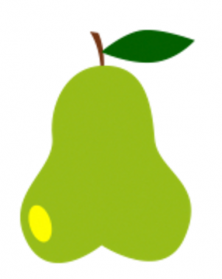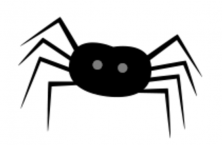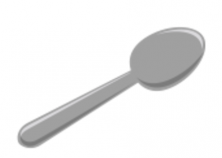Smartick is an advanced online program that teaches kids math and coding in only 15 min. a day

Jun14

# Learn How to Convert Mass Measures in the Metric System

Hello! Welcome again to the Smartick blog. In today’s post, we will look at mass measures.

The main unit that is used to measure the mass of an object in the metric system is the gram. There are other units to measure mass like ounces, very commonly used in English-speaking countries.

In the metric system, the gram is not the only measurement used. Just like length and capacity, mass also has its multiples and submultiples. The multiples are for expressing units larger than the gram and the submultiples are for expressing measures smaller than the gram.

Do you remember what the prefixes for the multiples and submultiples were?

• The multiples: kilo, hecto, deca
• The submultiples: a deci, centi, milli

Take a look at the following table of mass measures:There are many more units of measures of mass in the Metric System but the most used are:

• Kilogram
• Hectogram
• Decagram
• Gram
• Decigram
• Centigram
• Milligram

Do you know what the most appropriate unit is to measure the mass of a truck? And to measure the mass of a teaspoon of sugar? Let’s see what the mass of some objects are in order to know what we can actually measure with each unit.

### Mass Measures:A car has a mass of approximately 1500 kilogramsA pear has a mass of approximately 2 hectogramsA pencil has a mass of approximately 1 decagramA small spider has a mass of approximately 1 gramOne tablespoon of water has a mass of approximately 1 centigram and a small teaspoon of water has a mass of approximately 5 milligrams.A drop of water has a mass much smaller than a milligram, approximately 0.05 milligrams.

Have you enjoyed this post about measures of mass?  Don’t hesitate to share it with your friends and classmates so that they too can learn the measure of mass. Until next time!